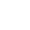/    2019-05-14

### related_name

``publish = ForeignKey(Blog, related_name='bookList')# 练习: 查询人民出版社出版过的所有书籍的名字与价格(一对多)# 反向查询 不再按表名:book,而是related_name:bookList    queryResult=Publish.objects　　　　　　　　　　　　　　.filter(name="人民出版社")　　　　　　　　　　　　　　.values_list("bookList__title","bookList__price")``

## 聚合查询与分组查询

### 聚合

aggregate(*args, **kwargs)

``# 计算所有图书的平均价格    >>> from django.db.models import Avg    >>> Book.objects.all().aggregate(Avg('price'))    {'price__avg': 34.35}``

aggregate()是QuerySet 的一个终止子句，意思是说，它返回一个包含一些键值对的字典。键的名称是聚合值的标识符，值是计算出来的聚合值。键的名称是按照字段和聚合函数的名称自动生成出来的。如果你想要为聚合值指定一个名称，可以向聚合子句提供它。

``>>> Book.objects.aggregate(average_price=Avg('price')){'average_price': 34.35}``

``>>> from django.db.models import Avg, Max, Min>>> Book.objects.aggregate(Avg('price'), Max('price'), Min('price')){'price__avg': 34.35, 'price__max': Decimal('81.20'), 'price__min': Decimal('12.99')}``

### 分组

``###################################－－单表分组查询－－#######################################################查询每一个部门名称以及对应的员工数emp:id  name age   salary    dep  alex  12   2000     销售部  egon  22   3000     人事部  wen   22   5000     人事部sql语句:select dep,Count(*) from emp group by dep;ORM:emp.objects.values("dep").annotate(c=Count("id")###################################－－多表分组查询－－###########################多表分组查询：查询每一个部门名称以及对应的员工数emp:id  name age   salary   dep_id  alex  12   2000       1  egon  22   3000       2  wen   22   5000       2depid   name    销售部   人事部emp－dep:id  name age   salary   dep_id   id   name   alex  12   2000       1      1    销售部  egon  22   3000       2      2    人事部  wen   22   5000       2      2    人事部sql语句:select dep.name,Count(*) from emp left join dep on emp.dep_id=dep.id group by dep.idORM:dep.objetcs.values("id").annotate(c=Count("emp")).values("name","c")``

``class Emp(models.Model):    name=models.CharField(max_length=32)    age=models.IntegerField()    salary=models.DecimalField(max_digits=8,decimal_places=2)    dep=models.CharField(max_length=32)    province=models.CharField(max_length=32)``

nnotate()为调用的QuerySet中每一个对象都生成一个独立的统计值（统计方法用聚合函数）。

### 查询练习

(1) 练习：统计每一个出版社的最便宜的书

``publishList=Publish.objects.annotate(MinPrice=Min("book__price"))for publish_obj in publishList:    print(publish_obj.name,publish_obj.MinPrice)``

annotate的返回值是querySet，如果不想遍历对象，可以用上valuelist：

``queryResult= Publish.objects　　　　　　　　　　　　.annotate(MinPrice=Min("book__price"))　　　　　　　　　　　　.values_list("name","MinPrice")print(queryResult)``
``'''SELECT "app01_publish"."name", MIN("app01_book"."price")  AS "MinPrice" FROM "app01_publish" LEFT  JOIN "app01_book" ON ("app01_publish"."nid" = "app01_book"."publish_id") GROUP BY "app01_publish"."nid", "app01_publish"."name", "app01_publish"."city", "app01_publish"."email" '''``

(2) 练习：统计每一本书的作者个数

``ret=Book.objects.annotate(authorsNum=Count('authors__name'))``

(3) 统计每一本以py开头的书籍的作者个数：

`` queryResult=Book.objects　　　　　　　　　　 .filter(title__startswith="Py")　　　　　　　　　 　.annotate(num_authors=Count('authors'))``

(4) 统计不止一个作者的图书：

``queryResult=Book.objects　　　　　　　　　　.annotate(num_authors=Count('authors'))　　　　　　　　　　.filter(num_authors__gt=1)``

(5) 根据一本图书作者数量的多少对查询集 QuerySet进行排序:

``Book.objects.annotate(num_authors=Count('authors')).order_by('num_authors')``

(6) 查询各个作者出的书的总价格:

``#   按author表的所有字段 group by    queryResult=Author.objects　　　　　　　　　　　　　　.annotate(SumPrice=Sum("book__price"))　　　　　　　　　　　　　　.values_list("name","SumPrice")    print(queryResult)``

## F查询与Q查询

### F查询

Django 提供 F() 来做这样的比较。F() 的实例可以在查询中引用字段，来比较同一个 model 实例中两个不同字段的值。

``# 查询评论数大于收藏数的书籍   from django.db.models import F   Book.objects.filter(commnetNum__lt=F('keepNum'))``

Django 支持 F() 对象之间以及 F() 对象和常数之间的加减乘除和取模的操作。

``# 查询评论数大于收藏数2倍的书籍    Book.objects.filter(commnetNum__lt=F('keepNum')*2)``

``Book.objects.all().update(price=F("price")+30)　``

### Q查询

filter() 等方法中的关键字参数查询都是一起进行“AND” 的。 如果你需要执行更复杂的查询（例如OR 语句），你可以使用Q 对象。

``from django.db.models import QQ(title__startswith='Py')``

Q 对象可以使用& 和| 操作符组合起来。当一个操作符在两个Q 对象上使用时，它产生一个新的Q 对象。

``bookList=Book.objects.filter(Q(authors__name="yuan")|Q(authors__name="egon"))``

``WHERE name ="yuan" OR name ="egon"``

``bookList=Book.objects.filter(Q(authors__name="yuan") & ~Q(publishDate__year=2017)).values_list("title")``

``bookList=Book.objects.filter(Q(publishDate__year=2016) | Q(publishDate__year=2017),                              title__icontains="python"                             )``(0)